# NormalizeDouble(Bid, Digits)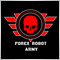28001

I want to get exact BID,ASK value with normalization. But i can not able to do that following most popular way.

`Print("Bid : " +Bid + "  Ask : "+Ask);`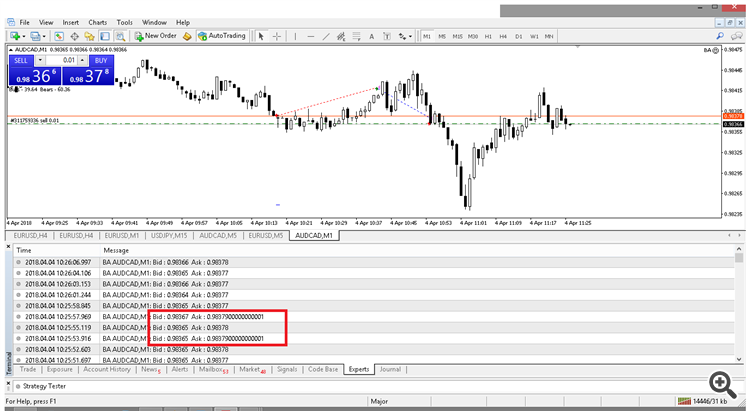Same result on after "NormalizeDouble"

```string Sbid1 = NormalizeDouble(Bid,Digits);
//double Dbid1 = StrToDouble(Sbid1);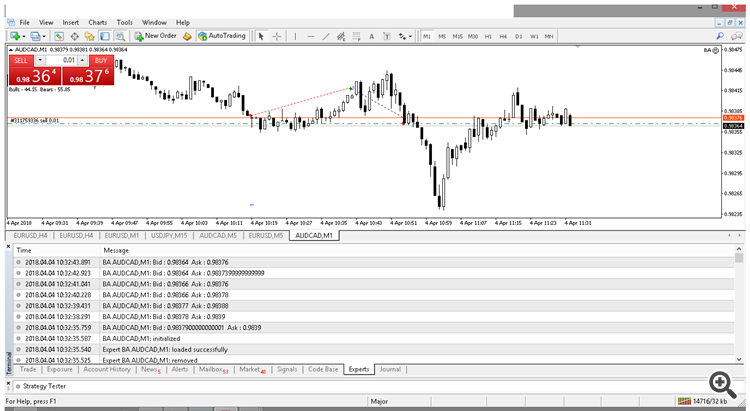In this case my symbol is AUDCAD. And i want to say When i put Take profit = 2,4,5 etc... it placed take profit level as 12pips from the order open price.

But when i put Take profit = 15,20,40,50 etc.... it placed correctly.

Why this happen? I read few posts about that. But i can not able to fix it. And i want to ask WHY IT NOT DOING BY MQL4 DEFAULT NORMALIZATION?

Is anyone help to me?

Thank you.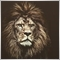9849

Thushara Dissanayake:

I want to get exact BID,ASK value with normalization. But i can not able to do that following most popular way.

Same result on after "NormalizeDouble"

In this case my symbol is AUDCAD. And i want to say When i put Take profit = 2,4,5 etc... it placed take profit level as 12pips from the order open price.

But when i put Take profit = 15,20,40,50 etc.... it placed correctly.

Why this happen? I read few posts about that. But i can not able to fix it. And i want to ask WHY IT NOT DOING BY MQL4 DEFAULT NORMALIZATION?

Is anyone help to me?

Thank you.

Try this Code,

```string Sbid1 = DoubleToStr(Bid,Digits);

Normally this happens.. It is even mentioned in the MQL4 Reference.28001

Lakshan Perera:

Try this Code,

Normally this happens.. It is even mentioned in the MQL4 Reference.

It is ok for Print(). But How For Order Send?

`ticket=OrderSend(Symbol(),OP_BUY,LotsOptimized(),Ask,3,0,(Ask+NDB(pips*Take_Profit)),"",MagicNumber,0,Green);`9849

For that just use NormalizeDouble(),

```TP_Level = NormalizeDouble((Ask+NDB(pips*Take_Profit),Digits);20228

Thushara Dissanayake: I want to get exact BID,ASK value with normalization.
Lakshan Perera: For that just use NormalizeDouble(),
Do NOT use NormalizeDouble, EVER. For ANY Reason. It's a kludge, don't use it. It's use is always wrong9849

whroeder1:

I am sorry why did you say "Do NOT use NormalizeDouble, EVER. For ANY Reason." . It is said in the MQL4 Reference to use NormalizeDouble() when calculating TP and SL levels.
I agree with other parts though(Lot Size etc.)38701

Lakshan Perera:

I am sorry why did you say "Do NOT use NormalizeDouble, EVER. For ANY Reason." . It is said in the MQL4 Reference to use NormalizeDouble() when calculating TP and SL levels.
I agree with other parts though(Lot Size etc.)

ea work fine for all pairs exept on YEN pairs

Alain Verleyen, 2017.09.29 20:19

Honestly I am tired to read this on the forum, not only WHRoeder post it almost every day, but now you start with the same too. The OP will not even understand why you write it.

There is no problem with NormalizeDouble(). It can be used, if done correctly. If you trade non-forex symbol it will not be sufficient to get a correct price.9849

Alain Verleyen:

Thanks, Yeah I understand the limitations of NormalizeDouble(), but I was confused why did he say not to use it "EVER For Any Reason".. This case we discussed it for AUDCAD(As said in the first post)20228

I said "it use is always wrong," and gave examples why. Post a use and I will (again) explain why it's wrong.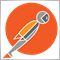2392

whroeder1:
I said "it use is always wrong," and gave examples why. Post a use and I will (again) explain why it's wrong.

Always wrong??? Please explain how/why this use is wrong, and also explain how your use of MathRound is correct given that it produces bugs in the way that you use it.

I wanted to add something to this conversation, since I recently learned that I was wrong about the use of NormalizeDouble. I originally discovered the error in my ways when user Amrali pointed out some errors in my CDouble library. As it turns out, the typically recommended algorithm for rounding to tick-size using the MathRound function produces errors.

`double error_prone_result = tick_size * round(price / tick_size);`

This is what amrali pointed out...

These errors always occur at edge numbers which terminate in 5 (like 1.12345).

For example CDouble::RoundToStep(1.700605, 0.00001) returns 1.70060, instead of the correct result 1.70061

The equation round(number / point) * point, should be corrected to round(number * power) / power, where both point and power are derived from the number of decimal digits you would like to round to.

Because the value of 1 point which is supposed = 0.00001, is actually encoded as 0.0000100000000000000008180305391403130954586231382563710 as a 64-bits double-precision floating point. This causes the final result from you rounding method, round(number / point) * point,  to drift from the correct result by 1 point (0.00001), very often.

In addition, in order to do a proper 'arithmetic' rounding (Midpoint Rounding away from zero), a good method is to add or subtract a half-epsilon as a correction. (This will offset any half-to-even rounding that has been applied by the processor, as mandated by the IEEE-754 specs, particularly at the midpoint edge cases).

The mql's NormalizeDouble() function handles all those issues correctly, you should use it to do proper 'arithmetic' rounding.

So what that means to everyone in this thread (whether you want to hate NormalizeDouble or not) is that if you are rounding to tick-size then you need to be using NormalizeDouble in your rounding methods.

`double rounded_tick = tick_size * NormalizeDouble(price / tick_size, 0);`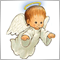3037

whroeder1:
I said "it use is always wrong," and gave examples why. Post a use and I will (again) explain why it's wrong.

As usually (in this point) you are wrong. You should stop posting this opinion every day. What is wrong with this code:

```double profitUSD=1234.56;
double profitEUR=NormalizeDouble(profitUSD/SymbolInfoDouble("EURUSD",SYMBOL_BID),2);```